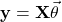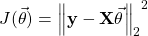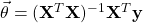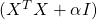2022-05-26
###### Understanding Ordinal and One-Hot Encodings for categorical features
2022-05-26

Many machine learning models demand that categorical features are converted to a format they can comprehend via a widely used feature engineering technique called one-hot encoding. Machines aren’t that smart.

A common convention after one-hot encoding is to remove one of the one-hot encoded columns from each categorical feature. For example, the feature sex containing values of male and female are transformed into the columns sex_male and sex_female, each containing binary values. Because using either of these columns provides sufficient information to determine a person’s sex, we can drop one of them.

In this post, we dive deep into the circumstances where this convention is relevant, necessary, or even prudent.

1. Preparing the data
2. Creating a linear regression model with ordinary least-squares
3. Making the normal equation usable again
4. Regularizing improves predictions and then some
5. Don’t bother dropping columns when regularizing
6. Skip dropping columns when using iterative numerical methods
7. Maybe just stop dropping columns altogether
8. Conclusions

## Preparing the data

Let’s generate a toy dataset with three variables; the third column serves as the target variable while the remaining are categorical features. Because we’re working with a continuous target variable, we’ll create a linear regression model.

# Load packages
import numpy as np
import pandas as pd

# Create training set
training_set = pd.DataFrame(
[
['apple', 'dog', 10],
['banana', 'cat', 4],
['pear', 'fish', 39],
['orange', 'dog', -12],
['apple', 'fish', 21],
['pear', 'cat', 53],
['apple', 'fish', -69]
],
columns=['var1', 'var2', 'var3']
)

training_set


We can use the pandas function get_dummies to perform one-hot encoding and generate the feature matrix X.

Let’s also add a bias term to X as a new column so that any model we create isn’t confined to passing through the origin.

# One-hot encode categorical features
X = pd.get_dummies(training_set[['var1', 'var2']])

X['bias'] = np.ones(X.shape)

# Display first three rows


Finally, let’s identify the target variable y.

# Extract target variable
y = training_set['var3']


## Creating a linear regression model with ordinary least-squares

In a linear regression model, we express the target variable y as a linear function of the features X and some unknown set of parameters θ:The simplest algorithm for finding this “line of best fit” is ordinary least-squares (OLS); it identifies θ that minimizes the sum of the squared residuals. Therefore, the objective function of OLS isNext, we have to solve the system of first-order partial differential equations ∂J/∂θ=0, which conveniently has a closed-form solution called the normal equation:Let’s apply the normal equation to identify the parameters of the OLS model.

# Compute parameters of OLS model
OLS_theta = np.linalg.inv(X.T @ X) @ (X.T @ y)

# Label parameters with feature names
pd.Series(OLS_theta, index=X.columns)
﻿
---------------------------------------------------------------------------
LinAlgError                               Traceback (most recent call last)
<ipython-input-4-d1b033489f2a> in <module>
1 # Compute parameters of OLS model
----> 2 OLS_theta = np.linalg.inv(X.T @ X) @ (X.T @ y)
3
4 # Label parameters with feature names
5 pd.Series(OLS_theta, index=X.columns)

~/miniconda3/envs/phoenix/lib/python3.7/site-packages/numpy/linalg/linalg.py in inv(a)
549     signature = 'D->D' if isComplexType(t) else 'd->d'
550     extobj = get_linalg_error_extobj(_raise_linalgerror_singular)
--> 551     ainv = _umath_linalg.inv(a, signature=signature, extobj=extobj)
552     return wrap(ainv.astype(result_t, copy=False))
553

~/miniconda3/envs/phoenix/lib/python3.7/site-packages/numpy/linalg/linalg.py in _raise_linalgerror_singular(err, flag)
95
96 def _raise_linalgerror_singular(err, flag):
---> 97     raise LinAlgError("Singular matrix")
98
99 def _raise_linalgerror_nonposdef(err, flag):

LinAlgError: Singular matrix


NumPy got angry because we tried to invert a singular matrix. Specifically,(the Gram matrix of X) was found to be singular, meaning it doesn’t have an inverse. In fact, the Gram matrix is invertible if and only if the columns of X are linearly independent.

Examining the columns of X, we see that

var1_apple = 1 – (var1_orange + var1_pear + var1_banana)

var2_cat = 1 – (var2_dog + var2_fish)

For any categorical feature, each one-hot encoded column can be expressed as a linear combination of the others—they’re perfectly correlated. Therefore, the columns of X are linearly dependent, which explains the error.

## Making the normal equation usable again

By dropping one of the one-hot encoded columns from each categorical feature, we ensure there are no “reference” columns—the remaining columns become linearly independent.

Let’s verify this works by implementing it; get_dummies even has a dedicated parameter drop_first.

# One-hot encode categorical features and drop first value column
X_dropped = pd.get_dummies(training_set[['var1', 'var2']], drop_first=True)

X_dropped['bias'] = np.ones(X.shape)

# Display first three rows


We see that var1_apple and var2_cat were dropped. Let’s reattempt to use the normal equation to identify the parameters of the OLS model.

# Compute parameters of OLS model
OLS_theta = np.linalg.inv(X_dropped.T @ X_dropped) @ (X_dropped.T @ y)

# Label parameters with feature names
pd.Series(OLS_theta, index=X_dropped.columns)

var1_banana    14.0
var1_orange   -22.0
var1_pear      63.0
var2_dog       20.0
var2_fish     -14.0
bias          -10.0
dtype: float64

Smooth sailing this time. Therefore, when using the normal equation to create an OLS model, you must drop one of the one-hot encoded columns from each categorical feature.

## Regularizing improves predictions and then some

OLS models are handy when we’d like to summarize linear trends for data we already have. When the goal is prediction, however, these models are seldom useful because of their numerous pitfalls. In particular, OLS models tend to generalize poorly to new data (aka overfitting).

To prevent overfitting, applying some form of regularization is a no-brainer. ℓ2 regularization involves adding a penalty term—square of the ℓ2 norm of θ to the objective function. Applying ℓ2 regularization to the OLS objective function yieldswhere α is a positive scalar hyperparameter that controls the degree of regularization (higher = more regularization).

We need to solve a new system of partial differential equations ∂J/∂θ=0; fortunately, it too has a closed-form solutionwhere II is an identity matrix with the same dimensions as the Gram matrix. Let’s identify the parameters of the ℓ2 regularized model using α=1.

def create_L2_reg_model(X, y, alpha):
"""
Generate a L2 regularized linear regression model.

This function uses the closed-form solution to compute the parameters of
an L2 regularized linear regression model.

Args:
X (DataFrame): table containing features
y (Series): table containing target variable
alpha (float): positive scalar controlling regularization strength
(higher = more regularization)

Returns:
theta (Series): table containing identified parameters of model

"""
# Compute identity matrix
I = np.identity((X.T @ X).shape)

# Compute parameters
theta = np.linalg.inv(X.T @ X + alpha * I) @ (X.T @ y)

# Label parameters with feature names
theta = pd.Series(theta, index=X.columns)

return theta

# Create L2 regularized model after dropping columns
create_L2_reg_model(X_dropped, y, alpha=1)

var1_banana     0.246537
var1_orange    -7.501385
var1_pear      32.678670
var2_dog       -0.504155
var2_fish     -13.049861
bias            3.506925
dtype: float64

A regularized model will generally perform better on new data than an OLS model. In practice, however, we’d tune the value of α using cross-validation to maximize model performance.

## Don’t bother dropping columns when regularizing

Having understood the benefits of regularization, let’s try to generate a ℓ2 regularized model with the closed-form solution but instead use the original one-hot encoded features prior to dropping any columns. We’ll probably run into the singular matrix error again.

# Create L2 regularized model using original one-hot encoded features
create_L2_reg_model(X, y, alpha=1)

var1_apple     -9.617518
var1_banana    -4.306569
var1_orange    -9.543066
var1_pear      27.564964
var2_cat        8.515328
var2_dog        2.988321
var2_fish      -7.405839
bias            4.097810
dtype: float64

Wait, shouldn’t NumPy have gotten angry? How were we still able to create a model? The answer is because, in the closed-form solution of the ℓ2 regularized model above, the matrixis almost surely nonsingular. Proof:

“Almost surely” is an expression from probability theory describing events that occur with P=1 within an infinitely large sample space. Therefore, as long as α isn’t the negative of an eigenvalue of, there exist infinitely many values of α that makenonsingular. Practically any perturbation of a singular matrix makes it nonsingular!

Consequently, if we apply the tiniest bit of regularization (whether it’s ℓ2, ℓ1, or elastic net), we can handle features that are perfectly correlated without removing any columns. Regularization also innately addresses the effects of multicollinearity—it’s pretty awesome.

But if you are regularizing, there’s no need to drop one of the one-hot encoded columns from each categorical feature—math’s got your back.

## Skip dropping columns when using iterative numerical methods

As elegant as they are, closed-form solutions are seldom utilized in practice. That’s because matrix inversion is stupidly expensive. The time complexity of inverting an n×nn×n matrix is O(n3) when using Gaussian elimination; more optimized algorithms can bring it down to about O(n2.4). Unless it has a few hundred columns (rarely the case with real-world datasets), you shouldn’t attempt to invert a matrix.

Instead of relying on a closed-form solution, we machine learning practitioners estimate parameters via some efficient iterative numerical method such as gradient descent. Because iterative numerical methods—with or without regularization—don’t involve matrix inversions, there’s no reason to drop one of the one-hot encoded columns from each categorical feature when using them.

## Maybe just stop dropping columns altogether

So far we’ve discussed a few situations where removing one of the one-hot encoded columns isn’t mandatory. However, dropping these columns can also have unforeseen, deleterious consequences.

Did you notice that the parameters between one-hot encoded features had different values depending on whether columns were removed or not? For example, when columns are dropped

θvar1_banana=−4.307 and θvar2_dog=2.988; otherwise, θvar1_banana=0.247 and θvar2_dog=−0.504. If we were planning to use these parameters to get a sense of feature importance, dropping columns would tell a whole other story!

Because we alter the model’s parameters by dropping one-hot encoded columns, we also change its predictions. What’s more alarming is that dropping a different column from each categorical feature yields an entirely new set of parameters.

For example, instead of var1_apple and var2_cat, let’s drop var1_banana and var2_dog from the one-hot encoded features.

# Drop different one-hot encoded columns from each categorical feature
X_dropped = X.drop(['var1_banana', 'var2_dog'], axis=1)

# Create L2 regularized model after dropping different set of columns
create_L2_reg_model(X_dropped, y, alpha=1)

var1_apple     -8.651452
var1_orange    -8.199170
var1_pear      28.286307
var2_cat        6.639004
var2_fish      -8.294606
bias            4.398340
dtype: float64

If we arrive at a different model depending on the particular set of columns removed, how do we pick the right model? There’s no good answer here—removing columns isn’t trivial. You’re better off staying objective and leaving one-hot encoded features alone.

## Conclusions

Feature engineering is the most important aspect of creating an effective model—you want to get it right. When dealing with categorical features, a common convention is to drop one of the one-hot encoded columns from each feature. Here we discovered this convention is only required when creating an OLS model with the normal equation.

However, a cornerstone of machine learning is to produce a highly predictive model; therefore, we rarely turn to OLS models and always apply regularization. Even if we were to create a ℓ2 regularized model with a closed-form solution, the gorgeous math behind regularization would lift the obligation of removing one-hot encoded columns.

Nevertheless, the normal equation and other closed-form solutions are seldom practical due to their computational cost. Instead, we machine learning practitioners prefer creating linear regression models using iterative numerical methods that don’t demand dropping one-hot encoded columns.

Finally, we found that dropping one-hot encoded columns tampers with a linear regression model’s parameters and predictions. We also end up with a distinct model depending on which set of columns we happened to drop.

In summary, we’ve uncovered one unlikely use case where removing one of the one-hot encoded from each categorical feature is crucial for creating a linear regression model, two common situations when it’s unnecessary, and two reasons why it’s perilous. I’ll leave it to you.

What about logistic regression? The same reasons actually apply to generalized linear models. There’s even less of a reason to drop one-hot encoded columns when using logistic regression because there is no known closed-form solution for identifying its parameters. We always rely on an iterative numerical method. That is unless your training set has two examples.

Side note: I recommend avoiding pandas’ get_dummies and switching to a more robust one-hot encoder, such as OneHotEncoder from scikit-learn—it’s designed to handle these frequent scenarios:

• A categorical feature containing values that appear in the test set but not the training set
• A categorical feature in the test set containing a subset of the total possible values

Notice how OneHotEncoder doesn’t let us drop one-hot encoded columns

Source:

https://inmachineswetrust.com/posts/drop-first-columns/##### Amir Masoud Sefidian
Machine Learning Engineer